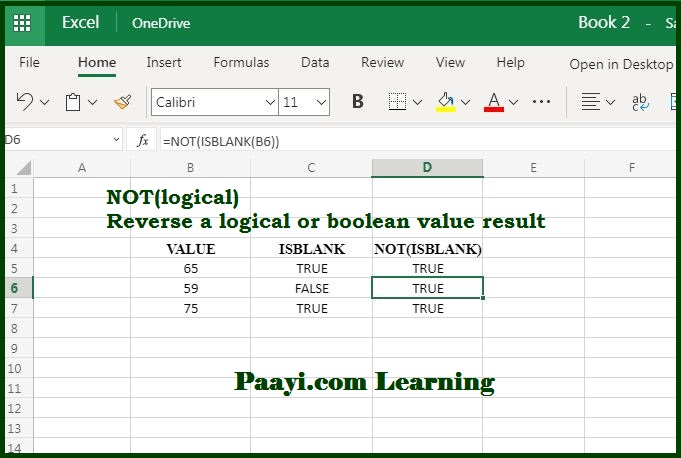# Learn How to Use Microsoft Excel NOT Function

Written by | 0 Comments | 737 Views

In this article, you will learn how to use the Microsoft Excel NOT function and its prime function in Microsoft Excel. You will also get to know the Microsoft Excel NOT function return value and syntax with the help of some examples.

Microsoft Excel NOT Function

The Microsoft Excel NOT function is used to reverse the arguments or results. That means the NOT function returns the reverse of boolean or logical value. If the result is TRUE,  NOT returns FLASE, and if the result is FALSE, the NOT function returns TRUE. So, the NOT function is used to reverse the logical value.

NOT Function Return Value

It will return the reverse of logical value.

NOT Function Syntax

=NOT (logical)

Where:

• logical: A value of logical expression which you can evaluate as TRUE or FALSE.

How to Use Microsoft Excel NOT Function?The NOT function is used when you wish to reverse a value or logical argument:

• If logical is FALSE, NOT returns TRUE.
• If logical is TRUE, it NOT returns FALSE.

It means the NOT function is used to reverse the way how another function is operated. Let us understand it more with the example given below:

=NOT(ISBLANK(A1))

If you use the above formula, the NOT function will return TRUE if cell A1 is blank. The NOT function allows you to create a formula that behaves like ISNOTBLANK, which is not otherwise possible in Microsoft Excels.